# Existence of solutions for a class of degenerate quasilinear elliptic equation in ${R}^{N}$ with vanishing potentials

## Abstract

We establish the existence of positive solution for the following class of degenerate quasilinear elliptic problem

where $-\mathcal{L}{u}_{ap}=-div\left({|x|}^{-ap}{|\mathrm{\nabla }u|}^{p-2}\mathrm{\nabla }u\right)$, $1, $-\mathrm{\infty }, $a\le e\le a+1$, $d=1+a-e$, and ${p}^{\ast }:={p}^{\ast }\left(a,e\right)=\frac{Np}{N-dp}$ denote the Hardy-Sobolev’s critical exponent, V is a bounded nonnegative vanishing potential and f has a subcritical growth at infinity. The technique used here is a truncation argument together with the variational approach.

MSC:35B09, 35J10, 35J20, 35J70.

## 1 Introduction

Consider the following degenerate quasilinear elliptic problem in ${R}^{N}$:

where $-\mathcal{L}{u}_{ap}=-div\left({|x|}^{-ap}{|\mathrm{\nabla }u|}^{p-2}\mathrm{\nabla }u\right)$, $1, $-\mathrm{\infty }, $a\le e\le a+1$, $d=1+a-e$, and ${p}^{\ast }:={p}^{\ast }\left(a,e\right)=\frac{Np}{N-dp}$ denote the Hardy-Sobolev’s critical exponent, $V:{R}^{N}\to R$ is a bounded, nonnegative and vanishing potential and $f:R\to R$ a continuous function with a subcritical growth at infinity. Here, ${\mathcal{D}}_{a}^{1,p}\left({R}^{N}\right)$ is the completion of the ${\mathcal{C}}_{0}^{\mathrm{\infty }}\left({R}^{N}\right)$ with the norm $|u|={\left({\int }_{{R}^{N}}{|x|}^{-ap}{|\mathrm{\nabla }u|}^{p}\phantom{\rule{0.2em}{0ex}}dx\right)}^{\frac{1}{p}}$. We impose the following hypotheses on f and V:

$f:R\to R$ is a continuous function verifying

(${f}_{1}$) ${lim sup}_{s\to {0}^{+}}\frac{sf\left(s\right)}{{|x|}^{-a{p}^{\ast }}{s}^{{p}^{\ast }}}<\mathrm{\infty }$, uniformly in x.

(${f}_{2}$) There exists $\alpha \in \left(p,{p}^{\ast }\right)$ such that ${lim sup}_{s\to \mathrm{\infty }}\frac{sf\left(s\right)}{{|x|}^{-a{p}^{\ast }}{s}^{\alpha }}<\mathrm{\infty }$, uniformly in x.

(${f}_{3}$) There exists $\theta >p$ such that $\theta F\left(s\right)\le sf\left(s\right)$, for all $s>0$.

$V:{R}^{N}\to R$ is a continuous function verifying

(${V}_{1}$) $V\left(x\right)\ge 0$, for all $x\in {R}^{N}$.

(${V}_{2}$) There are $\mathrm{\Lambda }>0$ and $\overline{r}>1$ such that ${inf}_{|x|>\overline{r}}V\left(x\right){|x|}^{\frac{{p}^{2}\left[N-p\left(a+1\right)\right]}{\left(p-1\right)\left(N-p\right)}}\ge \mathrm{\Lambda }$.

Remark 1.1 The conditions (${f}_{1}$) and (${f}_{2}$) imply the following:

(1)

Example 1.2 An example for the function f is given by

with $\lambda >{p}^{\ast }$ and α given by (${f}_{2}$).

By vanishing potential we mean a potential that vanish on some bounded domain or become very close to zero at infinity. An important example for a such potential is given by

with $\mathrm{\Lambda }>0$.

Consider first the case $a=0$, that is $-\mathcal{L}{u}_{ap}$ is the p-Laplacian operator, and the potential is bounded from below by a positive constant ${V}_{0}>0$.

Equations involving the p-Laplacian operator appear in many problems of nonlinear diffusion. Just to mention, in nonlinear optics, plasma physics, condensed matter physics and in modeling problems in non-Newtonian fluids. For more information on the physical background, we refer to .

For the case $p=2$, we cite , and references therein. In , in addition to the above assumptions, the authors consider a local condition, namely,

$\underset{x\in \overline{\mathrm{\Omega }}}{min}V<\underset{x\in \partial \mathrm{\Omega }}{min}V,$

where $\mathrm{\Omega }\subset {R}^{N}$ is a open bounded set, instead of the global condition imposed by Rabinowitz in . For $p\ne 2$, see .

Now, consider that V is the zero mass case, that is ${lim}_{|x|\to \mathrm{\infty }}V\left(x\right)=0$. When $p=2$, we cite  and the recent paper  by Alves and Souto.

Let us now consider the case $a\ne 0$ and the potential bounded from below by a positive constant ${V}_{0}>0$.

In this case, the equations arise in problems of existence of stationary waves for anisotropic Schrödinger equation (see ) and others problems (for example, see [6, 23]). We cite  for $p=2$; and [24, 25] for $p\ne 2$. For the case $V\equiv 0$, we cite , for $p=2$ and $a\ne 0$; and , for $p\ne 2$ and $a=0$.

The result presented here for $1 and $a\ne 0$ extends that one in  for $p=2$ and $a=0$. In , the presence of Hilbertian structure and some compact embeddings provide the convergence of the gradient. In the case studied here, with the absence of this structure, we do not obtain the convergence so directly. To overcome this problem, we use a result found in [28, 29], whose ideas come from [30, 31], when the domain is a smooth and bounded. In addition to this difficulty, there are others. For instance, in the present situation, our space is no longer Hilbert, which forces us to obtain new estimates. Since the problem involves singular terms, the estimates are more refined and for which the principal ingredient is the Caffarelli-Kohn-Nirenberg’s inequality (see ). Now we state the main result of this work.

Theorem 1.3 Suppose that V and f satisfy, respectively, (${V}_{1}$) and (${V}_{2}$) and (${f}_{1}$) to (${f}_{3}$). Then there is a constant${\mathrm{\Lambda }}^{\ast }={\mathrm{\Lambda }}^{\ast }\left({V}_{\mathrm{\infty }},\theta ,p,{c}_{0}\right)>0$such that the problem (P) has a positive solution, for all$\mathrm{\Lambda }\ge {\mathrm{\Lambda }}^{\ast }$, being${V}_{\mathrm{\infty }}$the maximum of the f in the ball of${R}^{N}$centered in the origin with radius 1.

In order to prove this theorem, we first build an auxiliary problem (AP), and then we solve the problem (AP) using variational methods. To finish, we show that the solution of (AP) is also a solution of (P). These steps are the content of the next three sections.

Hereafter, C is a positive constant which can change value in a sequence of inequalities. We denote ${B}_{R}={B}_{R}\left(0\right)$ the ball in ${R}^{N}$ centered in the origin with radius R. The weak () and strong (→) convergences are always taken as $n\to \mathrm{\infty }$ and ${\int }_{A}f$ means ${\int }_{A}f\left(x\right)\phantom{\rule{0.2em}{0ex}}dx$. The weighted ${L}^{p}$ spaces are denoted by ${L}_{\alpha }^{p}\left(A\right)=\left\{u:{R}^{N}\to R:{\int }_{A}{|x|}^{-\alpha }{|u|}^{p}<\mathrm{\infty }\right\}$. When $\alpha =0$, we denote ${\parallel \cdot \parallel }_{{L}^{p}\left(A\right)}$ the usual norm in ${L}^{p}\left(A\right)$, with $1\le p\le \mathrm{\infty }$. For $A={R}^{N}$, we use ${\parallel \cdot \parallel }_{p}$.

## 2 The auxiliary problem

As usual, since we are looking for positive solutions of problem (P), we set $f\left(t\right)=0$, for all $t\le 0$. The hypothesis (${V}_{1}$) allows us to consider the space

$E=\left\{u\in {\mathcal{D}}_{a}^{1,p}\left({R}^{N}\right):{\int }_{{R}^{N}}V{|x|}^{-a{p}^{\ast }}{|u|}^{p}<\mathrm{\infty }\right\},$

with norm

$\parallel u\parallel ={\left({\int }_{{R}^{N}}{|x|}^{-ap}{|\mathrm{\nabla }u|}^{p}+V{|x|}^{-a{p}^{\ast }}{|u|}^{p}\right)}^{\frac{1}{p}}.$

Associated to the problem (P), we define on E, the Euler-Lagrange functional

$I\left(u\right)={\int }_{{R}^{N}}{|x|}^{-ap}{|\mathrm{\nabla }u|}^{p}+V{|x|}^{-a{p}^{\ast }}{|u|}^{p}-{\int }_{{R}^{N}}F\left(u\right),$

being $F\left(s\right)={\int }_{0}^{s}f\left(t\right)\phantom{\rule{0.2em}{0ex}}dt$. From the assumptions on f, it follows that I is ${C}^{1}$ with Gâteaux derivative

${I}^{\prime }\left(u\right)v={\int }_{{R}^{N}}{|x|}^{-ap}{|\mathrm{\nabla }u|}^{p-2}\mathrm{\nabla }u\mathrm{\nabla }v+V{|x|}^{-a{p}^{\ast }}{|u|}^{p-2}uv-{\int }_{{R}^{N}}f\left(u\right)v,\phantom{\rule{1em}{0ex}}v\in E.$

To obtain solutions of problem (P), we introduce some truncation of the function f. Consider $k=\frac{p\theta }{\theta -p}>p$, $\overline{r}>1$ and define

(2)

Now we define the auxiliary problem:

(3)

Associated to the problem (AP), we define, on E, the Euler-Lagrange functional

$J\left(u\right)=\frac{1}{p}{\int }_{{R}^{N}}{|x|}^{-ap}{|\mathrm{\nabla }u|}^{p}+V{|x|}^{-a{p}^{\ast }}{|u|}^{p}-{\int }_{{R}^{N}}G\left(x,u\right)=\frac{1}{p}{\parallel u\parallel }^{p}-{\int }_{{R}^{N}}G\left(x,u\right),$

being $G\left(x,s\right)={\int }_{0}^{s}g\left(x,t\right)\phantom{\rule{0.2em}{0ex}}dt$. From the assumptions on f, it follows that J is ${C}^{1}$ with Gâteaux derivative

${J}^{\prime }\left(u\right)v={\int }_{{R}^{N}}{|x|}^{-ap}{|\mathrm{\nabla }u|}^{p-2}\mathrm{\nabla }u\mathrm{\nabla }v+V{|x|}^{-a{p}^{\ast }}{|u|}^{p-2}uv-{\int }_{{R}^{N}}g\left(x,u\right)v,\phantom{\rule{1em}{0ex}}v\in E.$

## 3 Solving the problem (AP)

In this section, we show that the problem (AP) has a least energy solution, but first we define some minimax levels. To begin with we set in the space ${\mathcal{D}}_{a}^{1,p}\left({B}_{1}\right)$, the norm $⦀u⦀={\left({\int }_{{B}_{1}}{|x|}^{-ap}{|\mathrm{\nabla }u|}^{p}+{V}_{\mathrm{\infty }}{|x|}^{-a{p}^{\ast }}{|u|}^{p}\right)}^{\frac{1}{p}}$ and we define the functional ${I}_{0}$ given by ${I}_{0}\left(u\right)=\frac{1}{p}{\int }_{{B}_{1}}{|x|}^{-ap}{|\mathrm{\nabla }u|}^{p}+{V}_{\mathrm{\infty }}{|x|}^{-a{p}^{\ast }}{|u|}^{p}-{\int }_{{B}_{1}}F\left(u\right)$. Here, ${\mathcal{D}}_{a}^{1,p}\left({B}_{1}\right)$ is the completion of the ${\mathcal{C}}_{0}^{\mathrm{\infty }}\left({B}_{1}\right)$ with the norm $|u|={\left({\int }_{{B}_{1}}{|x|}^{-ap}{|\mathrm{\nabla }u|}^{p}\right)}^{\frac{1}{p}}$.

Lemma 3.1 The functional${I}_{0}$has the mountain pass geometry, namely,

1. 1.

$\mathrm{\exists }{r}_{0},{\rho }_{0}>0$ such that ${I}_{0}\left(u\right)\ge {\rho }_{0}$ for $⦀u⦀={r}_{0}$.

2. 2.

$\mathrm{\exists }{e}_{0}\in {\mathcal{D}}_{a}^{1,p}\left({B}_{1}\right)$ such that $⦀{e}_{0}⦀\ge {r}_{0}$ and $J\left({e}_{0}\right)\le 0$.

Proof By using the growth of f given in Remark 1.1 and the Caffarelli-Kohn-Nirenberg’s inequality (see ), we get ${\int }_{{B}_{1}}F\left(u\right)\le {\int }_{{B}_{1}}{c}_{0}{|x|}^{-a{p}^{\ast }}{|u|}^{{p}^{\ast }}\le {c}_{0}⦀u{⦀}^{{p}^{\ast }}$, and hence ${I}_{0}\left(u\right)\ge \frac{1}{p}⦀u{⦀}^{p}-{c}_{0}⦀u{⦀}^{{p}^{\ast }}$. Since ${p}^{\ast }>p$, there exists ${r}_{0}$ such that ${\rho }_{0}:=\frac{1}{p}{r}_{0}^{p}-c{r}_{0}^{{p}^{\ast }}>0$. Thus, we have ${I}_{0}\left(u\right)\ge {\rho }_{0}$ for $⦀u⦀={r}_{0}$. By (${f}_{3}$), it follows that there exist $\theta >p$ and $C>0$ such that $F\left(s\right)\ge C{|s|}^{\theta }$. Now ${u}_{0}\in {\mathcal{D}}_{a}^{1,p}\left({B}_{1}\right)$ implies ${I}_{0}\left(t{u}_{0}\right)\le \frac{{t}^{p}}{p}⦀{u}_{0}{⦀}^{p}-{t}^{\theta }C{\int }_{{B}_{1}}{|{u}_{0}|}^{\theta }$. Since $\theta >p$, there exists a ${t}_{0}$ large enough such that, taking ${e}_{0}={t}_{0}{u}_{0}$, we have ${I}_{0}\left({e}_{0}\right)<0$ and $⦀{e}_{0}⦀\ge {r}_{0}$. □

Lemma 3.2 The functional J has the mountain pass geometry, namely,

1. 1.

$\mathrm{\exists }{r}_{1},{\rho }_{1}>0$ such that $J\left(u\right)\ge {\rho }_{1}$ for $\parallel u\parallel ={r}_{1}$.

2. 2.

$\mathrm{\exists }{e}_{1}\in E$ such that $\parallel {e}_{1}\parallel \ge {r}_{1}$ and $J\left({e}_{1}\right)\le 0$.

Proof From the definition of G, we have ${\int }_{{R}^{N}}G\left(x,u\right)\le {\int }_{{R}^{N}}F\left(u\right)$. Thus, like the previous lemma, we have $J\left(u\right)\ge {\rho }_{1}:=\frac{1}{p}{r}_{1}^{p}-c{r}_{1}^{{p}^{\ast }}>0$ for $\parallel u\parallel ={r}_{1}$. Take the same ${u}_{0}\in {\mathcal{D}}_{a}^{1,p}\left({B}_{1}\right)$ of the proof of the previous lemma. Thus, ${u}_{0}\in E$ and $G\left(x,u\right)=F\left(u\right)$. With the same argument, we have $J\left(t{u}_{0}\right)\le \frac{{t}^{p}}{p}{\parallel {u}_{0}\parallel }^{p}-{t}^{\theta }C{\int }_{{R}^{N}}{|{u}_{0}|}^{\theta }$. Since $\theta >p$, there exists a ${t}_{1}$ large enough such that, taking ${e}_{1}={t}_{1}{u}_{0}$, we have $J\left({e}_{1}\right)<0$ and $\parallel {e}_{1}\parallel \ge {r}_{1}$. □

Next, we are going to define two minimax levels, which will play an important role in our arguments. Note that is possible to take t such that $e=t{u}_{0}$ satisfies two previous lemmas. This allows to define the minimax levels c and $\overline{c}$ by

and

respectively. Since $J\left({u}_{0}\right)\le {I}_{0}\left({u}_{0}\right)$ in ${\mathcal{D}}_{a}^{1,p}\left({B}_{1}\right)$, we have $c\le \overline{c}$, by their definitions. Now using the above lemma together with the mountain pass theorem [, Theorem 2.2], we conclude that there exists a Palais-Smale sequence ((PS) sequence for short) $\left({u}_{n}\right)\subset E$ for J, i.e., $\left({u}_{n}\right)$ satisfies $J\left({u}_{n}\right)\to c$ and ${J}^{\prime }\left({u}_{n}\right)\to 0$.

Lemma 3.3 Suppose (${V}_{1}$) and (${f}_{1}$) to (${f}_{3}$) and let$\left({u}_{n}\right)\subset E$be a (PS) sequence for the functional J. Then$\left({u}_{n}\right)$is bounded in E.

Proof Define the set . In A, we have $G\left(x,u\right)=F\left(u\right)$. By using (${f}_{3}$), we conclude that there is $\theta >p$ such that $-G\left(x,u\right)+\frac{1}{\theta }ug\left(x,u\right)\ge 0$. So, we have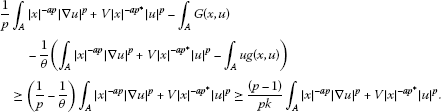Now consider the set . In B, we have ${\int }_{B}ug\left(x,u\right)>0$ and $G\left(x,u\right)=\frac{V}{pk}{|x|}^{-a{p}^{\ast }}{|u|}^{p}$. Then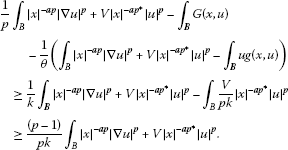Therefore, we get $J\left(u\right)-\frac{1}{\theta }{J}^{\prime }\left(u\right)u\ge \frac{\left(p-1\right)}{pk}{\parallel u\parallel }^{p}$. In particular, the above equation holds for the (PS) sequence $\left({u}_{n}\right)$ for J, and we have $J\left({u}_{n}\right)-\frac{1}{\theta }{J}^{\prime }\left({u}_{n}\right){u}_{n}\ge \frac{\left(p-1\right)}{pk}{\parallel {u}_{n}\parallel }^{p}$. On the other hand, we have $J\left({u}_{n}\right)\to c$, $\theta >p>1$ and ${J}^{\prime }\left({u}_{n}\right)\frac{{u}_{n}}{\parallel {u}_{n}\parallel }\to 0$, since ${J}^{\prime }\left({u}_{n}\right)\to 0$. Thus, we get $J\left({u}_{n}\right)-\frac{1}{\theta }{J}^{\prime }\left({u}_{n}\right){u}_{n}\le M+\frac{1}{\theta }\parallel {u}_{n}\parallel \le M+\parallel {u}_{n}\parallel$, for some constant $M>0$. Then we have $\frac{\left(p-1\right)}{pk}{\parallel {u}_{n}\parallel }^{p}\le M+\parallel {u}_{n}\parallel$, which can be rewritten as

$\parallel {u}_{n}\parallel \left(\left(p-1\right){\parallel {u}_{n}\parallel }^{p-1}-pk\right)\le pkM.$
(4)

Assuming $\parallel {u}_{n}\parallel \to \mathrm{\infty }$, equation (4) implies that $\left(p-1\right){\parallel {u}_{n}\parallel }^{p-1}-pk\to 0$. So $\parallel {u}_{n}\parallel \to {\left(\frac{pk}{p-1}\right)}^{\frac{1}{p-1}}$, which is a contradiction. Therefore, $\parallel {u}_{n}\parallel$ is bounded in E. □

Lemma 3.4 Suppose (${V}_{1}$) and (${f}_{1}$) to (${f}_{3}$). Then the functional J satisfies the Palais-Smale condition, i.e., every (PS) sequence has a convergent subsequence.

Proof Observe that, given the (PS) sequence $\left({u}_{n}\right)$, by Lemma 3.3, there exists $u\in E$ such that ${u}_{n}⇀u$, because E is reflexive space.a Thus, it is enough to show that $\parallel {u}_{n}\parallel \to \parallel u\parallel$. We divide this task in the four claims below.

1. 1.

Claim 1 ${\int }_{{R}^{N}}{u}_{n}g\left(x,{u}_{n}\right)\to {\int }_{{R}^{N}}ug\left(x,u\right)$.

2. 2.

Claim 2 ${\int }_{{R}^{N}}ug\left(x,{u}_{n}\right)\to {\int }_{{R}^{N}}ug\left(x,u\right)$.

3. 3.

Claim 3 ${\int }_{{R}^{N}}V{|x|}^{-a{p}^{\ast }}{|{u}_{n}|}^{p-2}{u}_{n}u\to {\int }_{{R}^{N}}V{|x|}^{-a{p}^{\ast }}{|u|}^{p}$.

4. 4.

Claim 4 ${\int }_{{R}^{N}}{|x|}^{-ap}{|\mathrm{\nabla }{u}_{n}|}^{p-2}\mathrm{\nabla }{u}_{n}\mathrm{\nabla }u\to {\int }_{{R}^{N}}{|x|}^{-ap}{|\mathrm{\nabla }u|}^{p}$.

Assuming Claims 1 to 4 for now, we proceed with the proof of lemma.

Since ${J}^{\prime }\left({u}_{n}\right){u}_{n}\to 0$, we have ${\int }_{{R}^{N}}{|x|}^{-ap}{|\mathrm{\nabla }{u}_{n}|}^{p}+V{|x|}^{-a{p}^{\ast }}{|{u}_{n}|}^{p}-{\int }_{{R}^{N}}{u}_{n}g\left(x,{u}_{n}\right)={o}_{n}\left(1\right)$, and by Claim 1, we get

$\underset{n\to \mathrm{\infty }}{lim sup}{\parallel {u}_{n}\parallel }^{p}={\int }_{{R}^{N}}ug\left(x,u\right).$
(5)

As ${J}^{\prime }\left({u}_{n}\right)u\to 0$, we get ${\int }_{{R}^{N}}{|x|}^{-ap}{|\mathrm{\nabla }{u}_{n}|}^{p-2}\mathrm{\nabla }{u}_{n}\mathrm{\nabla }u+V{|x|}^{-a{p}^{\ast }}{|{u}_{n}|}^{p-2}{u}_{n}u-{\int }_{{R}^{N}}ug\left(x,{u}_{n}\right)={o}_{n}\left(1\right)$. Passing the limit in the above equation and using Claims 2, 3 and 4, we get

${\parallel u\parallel }^{p}={\int }_{{R}^{N}}{|x|}^{-ap}{|\mathrm{\nabla }u|}^{p}+V{|x|}^{-a{p}^{\ast }}{|u|}^{p}={\int }_{{R}^{N}}ug\left(x,u\right).$
(6)

Using equations (5) and (6), we have $\parallel {u}_{n}\parallel \to \parallel u\parallel$. □

In order to prove the claims, for a given $ϵ>0$, we choose r satisfying, the following two conditions:

1. 1.

$max\left\{{\int }_{{B}_{2r}\setminus {B}_{r}}{|x|}^{-a{p}^{\ast }}{|u|}^{{p}^{\ast }},{\int }_{{B}_{2r}^{c}}V{|x|}^{-a{p}^{\ast }}{|u|}^{p}\right\}\le ϵ$.

2. 2.

$\eta ={\eta }_{r}\in {\mathcal{C}}_{0}^{\mathrm{\infty }}\left({B}_{r}^{c}\right)$ is such that $\eta \equiv 1$ in ${B}_{2r}^{c}$ and $0\le \eta \le 1$, $|\mathrm{\nabla }\eta |\le \frac{2}{{r}^{d}}$, for all $x\in {R}^{N}$.

Observe that condition 1 follows by integrability of ${|x|}^{-a{p}^{\ast }}{|u|}^{{p}^{\ast }}$ and $V{|x|}^{-a{p}^{\ast }}{|u|}^{p}$. Note that, when one of these conditions holds for some ${r}_{0}$, it also verifies for every $r\ge {r}_{0}$. Thus, we can choose an r that satisfies both conditions.

Remark 3.5 From now on, we consider the function g defined in (2) with $\overline{r}=r$ satisfying conditions 1 and 2 above. From the growth of g and the choice of r, we conclude:

1. 1.

$g\left(x,t\right)=f\left(t\right)$, $G\left(x,t\right)=F\left(t\right)$ in ${B}_{r}$;

2. 2.

$g\left(x,t\right)\le \frac{V}{k}{|x|}^{-a{p}^{\ast }}{|t|}^{p-2}t$, $G\left(x,t\right)\le \frac{V}{pk}{|x|}^{-a{p}^{\ast }}{|t|}^{p}$ in ${B}_{r}^{c}$;

3. 3.

${\int }_{{B}_{2r}^{c}}|ug\left(x,u\right)|\le {\int }_{{B}_{2r}^{c}}|u|\frac{V}{k}{|x|}^{-a{p}^{\ast }}{|u|}^{p-1}\le \frac{1}{k}{\int }_{{B}_{2r}^{c}}V{|x|}^{-a{p}^{\ast }}{|u|}^{p}<ϵ$.

### Verification of claims

In all proofs, except for Claim 4, we consider separately integration in ${B}_{2r}$ and ${B}_{2r}^{c}$.

Claim 1: In ${B}_{2r}$, by combining the dominated convergence theorem with the compact embedding of E in ${L}_{\alpha }^{r}\left({B}_{2r}\right)$, $1\le r<{p}^{\ast }$ and $\alpha <\left(a+1\right)r+N\left(1-\frac{r}{p}\right)$ (see [, Theorem 2.1]), we obtain ${\int }_{{B}_{2r}}{u}_{n}g\left(x,{u}_{n}\right)\to {\int }_{{B}_{2r}}ug\left(x,u\right)$. On the other hand, when integrating in ${B}_{2r}^{c}$ we do not have the compact embedding of E in ${L}_{\alpha }^{r}\left({B}_{2r}^{c}\right)$. In this case, we first estimate ${\int }_{{B}_{2r}^{c}}{|x|}^{-ap}{|\mathrm{\nabla }{u}_{n}|}^{p}+V{|x|}^{-a{p}^{\ast }}{|{u}_{n}|}^{p}$, by using the cut-off function η defined before. As $\left(\eta {u}_{n}\right)$ is also bounded we have ${J}^{\mathrm{\prime }}\left({u}_{n}\right)\left(\eta {u}_{n}\right)\to 0$, namely,

${\int }_{{R}^{N}}{|x|}^{-ap}{|\mathrm{\nabla }{u}_{n}|}^{p-2}\mathrm{\nabla }{u}_{n}\mathrm{\nabla }\left(\eta {u}_{n}\right)+V{|x|}^{-a{p}^{\ast }}{|{u}_{n}|}^{p-2}{u}_{n}\eta {u}_{n}={o}_{n}\left(1\right)+{\int }_{{R}^{N}}\eta g\left(x,{u}_{n}\right){u}_{n}.$
(7)

Since $\eta \equiv 0$ in ${B}_{r}$, equation (7) holds in ${B}_{r}^{c}$. Adding to this, the fact that $g\left(x,t\right)\le \frac{V}{k}{|x|}^{-a{p}^{\ast }}{|t|}^{p-2}t$ in ${B}_{r}^{c}$ we have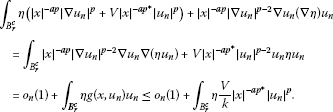From this, we obtainThus, we have(8)

Using the boundedness of $\left({u}_{n}\right)$ in E, i.e., $\parallel {u}_{n}\parallel \le C$, strong convergence of $\left({u}_{n}\right)$ in ${L}_{ap}^{p}\left({B}_{2r}\setminus {B}_{r}\right)$ and Hölder’s inequality we conclude that

$\begin{array}{rcl}\underset{n\to \mathrm{\infty }}{lim sup}{\int }_{{B}_{2r}\setminus {B}_{r}}{|x|}^{-ap}|{u}_{n}|{|\mathrm{\nabla }{u}_{n}|}^{p-1}& \le & C{\left({\int }_{{B}_{2r}\setminus {B}_{r}}{|x|}^{-ap}{|u|}^{p}\right)}^{\frac{1}{p}}\\ \le & C{\omega }_{N}^{\frac{d}{N}}{\left(2r\right)}^{d}{\left({\int }_{{B}_{2r}\setminus {B}_{r}}{|x|}^{-a{p}^{\ast }}{|u|}^{{p}^{\ast }}\right)}^{\frac{1}{{p}^{\ast }}},\end{array}$
(9)

where ${\omega }_{N}$ is the volume of the unit sphere in ${R}^{N}$. Therefore, given $ϵ>0$, by the choice of r and by equations (9) and (8), we infer thatHence,

$\underset{n\to \mathrm{\infty }}{lim sup}{\int }_{{B}_{2r}^{c}}{|x|}^{-ap}{|\mathrm{\nabla }{u}_{n}|}^{p}+V{|x|}^{-a{p}^{\ast }}{|{u}_{n}|}^{p}=0.$
(10)

Using this fact, we conclude that

$\begin{array}{rcl}\underset{n\to \mathrm{\infty }}{lim sup}{\int }_{{B}_{2r}^{c}}{u}_{n}g\left(x,{u}_{n}\right)& \le & \underset{n\to \mathrm{\infty }}{lim sup}\frac{1}{k}{\int }_{{B}_{2r}^{c}}V{|x|}^{-a{p}^{\ast }}{|{u}_{n}|}^{p}\\ \le & \underset{n\to \mathrm{\infty }}{lim sup}\frac{1}{k}{\int }_{{B}_{2r}^{c}}{|x|}^{-ap}{|\mathrm{\nabla }{u}_{n}|}^{p}+V{|x|}^{-a{p}^{\ast }}{|{u}_{n}|}^{p}=0.\end{array}$

Now, using Remark 3.5, we have ${lim sup}_{n\to \mathrm{\infty }}{\int }_{{B}_{2r}^{c}}|{u}_{n}g\left(x,{u}_{n}\right)-ug\left(x,u\right)|=0$.

Therefore, ${\int }_{{B}_{2r}^{c}}{u}_{n}g\left(x,{u}_{n}\right)\to {\int }_{{B}_{2r}^{c}}ug\left(x,u\right)$ and the proof of Claim 1 is completed.

Claim 2: The proof of this fact is made as in the proof of Claim 1.

Claim 3: In ${B}_{2r}$ the proof proceeds like in Claim 1. For integration in ${B}_{2r}^{c}$, we estimate the value of ${\int }_{{B}_{2r}}V{|x|}^{-a{p}^{\ast }}{|{u}_{n}|}^{p-2}{u}_{n}u$ by using the Hölder’s inequality

$\begin{array}{rcl}{\int }_{{B}_{2r}^{c}}V{|x|}^{-a{p}^{\ast }}{|{u}_{n}|}^{p-2}{u}_{n}u& \le & {\left({\int }_{{B}_{2r}^{c}}V{|x|}^{-a{p}^{\ast }}{|u|}^{p}\right)}^{\frac{1}{p}}{\left({\int }_{{B}_{2r}^{c}}V{|x|}^{-a{p}^{\ast }}{|{u}_{n}|}^{p}\right)}^{\frac{1}{{p}^{\mathrm{\prime }}}}\\ \le & C{\left({\int }_{{B}_{2r}^{c}}V{|x|}^{-a{p}^{\ast }}{|{u}_{n}|}^{p}\right)}^{\frac{1}{{p}^{\mathrm{\prime }}}}\\ \le & C{\left({\int }_{{B}_{2r}^{c}}{|x|}^{-ap}{|\mathrm{\nabla }{u}_{n}|}^{p}+V{|x|}^{-a{p}^{\ast }}{|{u}_{n}|}^{p}\right)}^{\frac{1}{{p}^{\mathrm{\prime }}}}.\end{array}$
(11)

Using equations (10) and (11), we have

$\underset{n\to \mathrm{\infty }}{lim sup}{\int }_{{B}_{2r}^{c}}V{|x|}^{-a{p}^{\ast }}{|{u}_{n}|}^{p-2}{u}_{n}u\le \underset{n\to \mathrm{\infty }}{lim sup}C{\left({\int }_{{B}_{2r}^{c}}{|x|}^{-ap}{|\mathrm{\nabla }{u}_{n}|}^{p}+V{|x|}^{-a{p}^{\ast }}{|{u}_{n}|}^{p}\right)}^{\frac{1}{{p}^{\mathrm{\prime }}}}=0.$

From this fact, we get ${\int }_{{B}_{2r}^{c}}V{|x|}^{-a{p}^{\ast }}{|{u}_{n}|}^{p-2}{u}_{n}u\to {\int }_{{B}_{2r}^{c}}V{|x|}^{-a{p}^{\ast }}{|u|}^{p}$ and the proof of this claim is completed.

Claim 4: Let ${p}^{\mathrm{\prime }}$ be dual of p. Since $u\in {\mathcal{D}}_{a}^{1,p}\left({R}^{N}\right)=\left\{u:{R}^{N}\to {R}^{N}:{|x|}^{-a}u\in {L}^{{p}^{\ast }}\left({R}^{N}\right)\phantom{\rule{0.5em}{0ex}}\text{and}\phantom{\rule{0.5em}{0ex}}{|x|}^{-a}\mathrm{\nabla }u\in {L}^{p}\left({R}^{N}\right)\right\}$,b we see that $h={|x|}^{-a}|\mathrm{\nabla }u|\in {L}^{p}\left({R}^{N}\right)$ and ${w}_{n}={|x|}^{-a\left(p-1\right)}{|\mathrm{\nabla }{u}_{n}|}^{p-2}\mathrm{\nabla }{u}_{n}\in {L}^{{p}^{\mathrm{\prime }}}\left({R}^{N}\right)$. As $\parallel {u}_{n}\parallel$ is bounded, we conclude that ${\parallel {w}_{n}\parallel }_{{L}^{{p}^{\mathrm{\prime }}}\left({R}^{N}\right)}$ is also bounded. Moreover, $\left({u}_{n}\right)$ satisfies the hypothesis of Lemma 3.6, below. So that we have $\mathrm{\nabla }{u}_{n}\to \mathrm{\nabla }u$ a.e. $x\in {R}^{N}$, which give us ${w}_{n}\to w={|x|}^{-a\left(p-1\right)}{|\mathrm{\nabla }u|}^{p-2}\mathrm{\nabla }u$ a.e. $x\in {R}^{N}$. Using a theorem [, Theorem 13.44], we conclude that ${w}_{n}⇀w$ in ${L}^{{p}^{\mathrm{\prime }}}\left({R}^{N}\right)$. Thus, we have ${\int }_{{R}^{N}}{w}_{n}h\to {\int }_{{R}^{N}}wh$, and hence ${\int }_{{R}^{N}}{|x|}^{-ap}{|\mathrm{\nabla }{u}_{n}|}^{p-2}\mathrm{\nabla }{u}_{n}\mathrm{\nabla }u\to {\int }_{{R}^{N}}{|x|}^{-ap}{|\mathrm{\nabla }u|}^{p}$.

Lemma 3.6 Let E and J be respectively the space and functional defined in Section  2. Let$\left({u}_{n}\right)\subset E$a bounded sequence such that${u}_{n}⇀u$in E and${J}^{\mathrm{\prime }}\left({u}_{n}\right)\to 0$. Then, passing to a subsequence if necessary, we have$\mathrm{\nabla }{u}_{n}\to \mathrm{\nabla }u$, a.e. $x\in {R}^{N}$.

The proof of this lemma follows using the same ideas made in  and , for a bounded domain. It can be found in [, Claim 1] and [, Lemma 1].

Using Lemmas 3.2, 3.3, 3.4 and the mountain pass theorem, in [, Theorem 2.4], we conclude that there exists $u\in E$ which is a critical point for the functional J, in the minimax level c. Moreover, u is the least energy solution to the problem (AP).

## 4 The solution of (AP) is solution of (P)

Now, our aim is to show that the solution found in the previous section is also a solution of the problem (P). It is sufficient to verify $f\left(u\right)\le \frac{V}{k}{|x|}^{-a{p}^{\ast }}{|u|}^{p-2}u$, for all $x\in {B}_{r}^{c}$.

Lemma 4.1 Any least energy solution u of (AP) satisfies the estimate${\parallel u\parallel }^{p}\le \frac{pk\overline{c}}{p-1}$.

Proof Since u is a critical point in the minimax level $c\le \overline{c}$, by Lemma 3.3, we have $\frac{\left(p-1\right)}{pk}{\parallel u\parallel }^{p}\le J\left(u\right)-\frac{1}{\theta }{J}^{\prime }\left(u\right)u=c\le \overline{c}$. So that ${\parallel u\parallel }^{p}\le \frac{pk\overline{c}}{p-1}$. □

Remark 4.2 The constant $\frac{pk\overline{c}}{p-1}$ depends only on ${V}_{\mathrm{\infty }}$, θ and f.

Lemma 4.3 Let h be such that${|x|}^{-a{p}^{\ast }}{|h|}^{q}$is integrable, with$pq>N$. Consider$H:{R}^{N}×R\to R$, and$b:{R}^{N}\to R$nonnegative and continuous functions such that$|H\left(x,s\right)|\le h\left(x\right){|x|}^{-a{p}^{\ast }}{|s|}^{p-1}$, for all$s>0$. Let$v\in E$be a weak solution of (AP 2):

$-\mathcal{L}{v}_{ap}+b{|x|}^{-a{p}^{\ast }}{|v|}^{p-2}v=H\left(x,v\right)\phantom{\rule{1em}{0ex}}\mathit{\text{in}}\phantom{\rule{0.5em}{0ex}}{R}^{N}.$

Then there exists a constant$M=M\left(q,{\parallel h\parallel }_{{L}_{a{p}^{\ast }}^{q}\left({R}^{N}\right)}\right)>0$such that${\parallel v\parallel }_{\mathrm{\infty }}\le M{\parallel {|x|}^{-a}v\parallel }_{{p}^{\ast }}$.

Proof Given $m\in N$ and $\beta >1$, set ${A}_{m}=\left\{x\in {R}^{N}:{|v|}^{\beta -1}\le m\right\}$, ${B}_{m}={R}^{N}\setminus {A}_{m}$ and

Thus

Then ${v}_{m}\in E$ and using it as the test function in (AP 2). we have

${\int }_{{R}^{N}}{|x|}^{-ap}{|\mathrm{\nabla }v|}^{p-2}\mathrm{\nabla }v\mathrm{\nabla }{v}_{m}+b{|x|}^{-a{p}^{\ast }}{|v|}^{p-2}v{v}_{m}={\int }_{{R}^{N}}H\left(x,v\right){v}_{m}.$
(12)

By definition of ${v}_{m}$, we get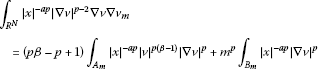(13)

and

${\int }_{{R}^{N}}b{|x|}^{-a{p}^{\ast }}{|v|}^{p-2}v{v}_{m}={\int }_{{A}_{m}}b{|x|}^{-a{p}^{\ast }}{|v|}^{p\beta }+{m}^{p}{\int }_{{B}_{m}}b{|x|}^{-a{p}^{\ast }}{|v|}^{p}>0.$
(14)

Using equations (13) and (14), we have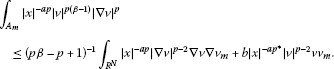(15)

Putting

we have

Thus,

${\int }_{{R}^{N}}{|x|}^{-ap}{|\mathrm{\nabla }{w}_{m}|}^{p}={\beta }^{p}{\int }_{{A}_{m}}{|x|}^{-ap}{|v|}^{p\left(\beta -1\right)}{|\mathrm{\nabla }v|}^{p}+{m}^{p}{\int }_{{B}_{m}}{|x|}^{-ap}{|\mathrm{\nabla }v|}^{p}.$
(16)

Now, taking into account (14), we get

$\begin{array}{rcl}{\int }_{{R}^{N}}b{|x|}^{-a{p}^{\ast }}{|{w}_{m}|}^{p}& =& {\int }_{{A}_{m}}b{|x|}^{-a{p}^{\ast }}{|v|}^{p\beta }+{m}^{p}{\int }_{{B}_{m}}b{|x|}^{-a{p}^{\ast }}{|v|}^{p}\\ =& {\int }_{{R}^{N}}b{|x|}^{-a{p}^{\ast }}{|v|}^{p-2}v{v}_{m}.\end{array}$
(17)

Using (17), (13) and (16), we have(18)

Combining (18), (15) and (12), we obtain

${\int }_{{R}^{N}}{|x|}^{-ap}{|\mathrm{\nabla }{w}_{m}|}^{p}+b{|x|}^{-a{p}^{\ast }}{|{w}_{m}|}^{p}\le {\beta }^{p}{\int }_{{R}^{N}}H\left(x,v\right){v}_{m}.$
(19)

Let S be the best constant for inequality ${\left({\int }_{{R}^{N}}{|x|}^{-a{p}^{\ast }}{|u|}^{{p}^{\ast }}\right)}^{\frac{p}{{p}^{\ast }}}\le S{\int }_{{R}^{N}}{|x|}^{-ap}{|\mathrm{\nabla }u|}^{p}$, for all $u\in {\mathcal{D}}_{a}^{1,p}\left({R}^{N}\right)$. (See .) By the inequality (19), the boundedness of H and the definitions of ${v}_{m}$ and S, we get ${\left({\int }_{{A}_{m}}{|x|}^{-a{p}^{\ast }}{|{w}_{m}|}^{{p}^{\ast }}\right)}^{\frac{p}{{p}^{\ast }}}\le S{\beta }^{p}{\int }_{{R}^{N}}h\left(x\right){|x|}^{-a{p}^{\ast }}{|v|}^{p\beta }$. Since $|{w}_{m}|={|v|}^{\beta }$ in ${A}_{m}$, we have

${\left({\int }_{{A}_{m}}{|x|}^{-a{p}^{\ast }}{|v|}^{{p}^{\ast }\beta }\right)}^{\frac{p}{{p}^{\ast }}}\le S{\beta }^{p}{\int }_{{R}^{N}}h\left(x\right){|x|}^{-a{p}^{\ast }}{|v|}^{p\beta }.$

Making $m\to \mathrm{\infty }$ and using the monotone convergence theorem, we get

${\left({\int }_{{R}^{N}}{|x|}^{-a{p}^{\ast }}{|v|}^{{p}^{\ast }\beta }\right)}^{\frac{p}{{p}^{\ast }}}\le S{\beta }^{p}{\int }_{{R}^{N}}h\left(x\right){|x|}^{-a{p}^{\ast }}{|v|}^{p\beta }.$

Since $pq>N$, $\sigma =\frac{N}{{q}_{1}\left(N-p\right)}>1$. Thus, we can consider $\beta ={\sigma }^{j}$ for $j=1,2,3,\dots$ and, using the Hölder’s inequality, we have

$\begin{array}{rcl}{\parallel {|x|}^{-\frac{a}{{\sigma }^{j}}}v\parallel }_{{p}^{\ast }{\sigma }^{j}}^{p{\sigma }^{j}}& \le & S{\sigma }^{jp}{\int }_{{R}^{N}}h\left(x\right){|x|}^{-a{p}^{\ast }}{|v|}^{p{\sigma }^{j}}\\ \le & S{\sigma }^{jp}{\left[{\int }_{{R}^{N}}{|x|}^{-a{p}^{\ast }}{|h\left(x\right)|}^{q}\right]}^{\frac{1}{q}}{\left[{\int }_{{R}^{N}}|{|x|}^{-\frac{a}{{\sigma }^{j-1}}}|v|{|}^{p{\sigma }^{j}{q}_{1}}\right]}^{\frac{1}{{q}_{1}}}\\ \le & {M}_{0}{\sigma }^{jp}{\left[{\int }_{{R}^{N}}|{|x|}^{-\frac{a}{{\sigma }^{j-1}}}|v|{|}^{p{\sigma }^{j}{q}_{1}}\right]}^{\frac{1}{{q}_{1}}}\le {M}_{0}{\sigma }^{jp}{\parallel {|x|}^{-\frac{a}{{\sigma }^{j-1}}}v\parallel }_{p{\sigma }^{j}{q}_{1}}^{p{\sigma }^{j}},\end{array}$

being ${M}_{0}=S{\left[{\int }_{{R}^{N}}{|x|}^{-a{p}^{\ast }}{|h\left(x\right)|}^{q}\right]}^{\frac{1}{q}}$. Note that ${M}_{0}$ is independent of j. Thus, we get

(20)

For $j=1$ and $j=2$, we have $p\sigma {q}_{1}={p}^{\ast }$ and $p{\sigma }^{2}{q}_{1}={p}^{\ast }\sigma$. Applying this in (20), we have

$\begin{array}{r}{\parallel {|x|}^{-\frac{a}{\sigma }}v\parallel }_{{p}^{\ast }\sigma }\le {M}_{0}^{\frac{1}{p\sigma }}{\sigma }^{\frac{1}{\sigma }}{\parallel {|x|}^{-a}v\parallel }_{{p}^{\ast }}\phantom{\rule{1em}{0ex}}\text{and}\\ {\parallel {|x|}^{-\frac{a}{{\sigma }^{2}}}v\parallel }_{{p}^{\ast }{\sigma }^{2}}\le {M}_{0}^{\frac{1}{p{\sigma }^{2}}}{\sigma }^{\frac{2}{{\sigma }^{2}}}{\parallel {|x|}^{-\frac{a}{\sigma }}v\parallel }_{{p}^{\ast }\sigma }.\end{array}$

By iterating, we have

${\parallel {|x|}^{-\frac{a}{{\sigma }^{2}}}v\parallel }_{{p}^{\ast }{\sigma }^{2}}\le {\sigma }^{\frac{1}{\sigma }+\frac{2}{{\sigma }^{2}}}{M}_{0}^{\frac{1}{p}\left(\frac{1}{\sigma }+\frac{1}{{\sigma }^{2}}\right)}{\parallel {|x|}^{-a}v\parallel }_{{p}^{\ast }}.$

Thus, we get

Then we can say that, for all $t\ge {p}^{\ast }$, we have

${\parallel v\parallel }_{t}\le {\sigma }^{\frac{\sigma }{{\left(\sigma -1\right)}^{2}}}{M}_{0}^{\frac{1}{p\left(\sigma -1\right)}}{\parallel {|x|}^{-a}v\parallel }_{{p}^{\ast }}.$

Putting $M:={\sigma }^{\frac{\sigma }{{\left(\sigma -1\right)}^{2}}}{M}_{0}^{\frac{1}{p\left(\sigma -1\right)}}$, we get

${\parallel v\parallel }_{\mathrm{\infty }}=\underset{t\to \mathrm{\infty }}{lim}{\parallel v\parallel }_{t}\le \underset{t\to \mathrm{\infty }}{lim}M{\parallel {|x|}^{-a}v\parallel }_{{p}^{\ast }}=M{\parallel {|x|}^{-a}v\parallel }_{{p}^{\ast }}.$

As σ depends on q, we have, by definition of M, that $M=M\left(q,{\parallel h\parallel }_{{L}_{a{p}^{\ast }}^{q}\left({R}^{N}\right)}\right)>0$. □

Remark 4.4 In the previous lemma, the constant M does not depend on the potential b of the problem (AP 2).

Lemma 4.5 There exists a constant${M}_{1}>0$such that${\parallel u\parallel }_{\mathrm{\infty }}\le {M}_{1}$, for all u positive solution of (AP).

Proof Take r from the definition of g and define and . Now define H and b by

We will show that, if u is solution of (AP), then u is weak solution of (AP 2).

In particular, we recall that $H\left(x,u\right)=f\left(u\right)=g\left(x,u\right)$, in A, and $g\left(x,u\right)=\frac{V}{k}{|x|}^{-a{p}^{\ast }}{|u|}^{p-2}u$, in B. Using the fact that ${R}^{N}$ is the disjoint union of A and B and the definitions above, for a given $\varphi \in E$, we write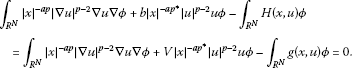Hence, u verifies ${\int }_{{R}^{N}}{|x|}^{-ap}{|\mathrm{\nabla }u|}^{p-2}\mathrm{\nabla }u\mathrm{\nabla }\varphi +b{|x|}^{-a{p}^{\ast }}{|u|}^{p-2}u\varphi ={\int }_{{R}^{N}}H\left(x,u\right)\varphi$, for all $\varphi \in E$. From (${f}_{1}$) and (${f}_{2}$), we obtain $|f\left(s\right)|\le {c}_{0}{|x|}^{-a{p}^{\ast }}{|s|}^{\alpha -1}$, for all $s>0$, with $\alpha \in \left(p,{p}^{\ast }\right)$. From the definition of H, it follows that $|H\left(x,u\right)|\le |f\left(u\right)|\le h\left(x\right){|x|}^{-a{p}^{\ast }}{|u|}^{p-1}$, with $h\left(x\right)={c}_{0}{|u|}^{\alpha -p}$. Taking $q=\frac{{p}^{\ast }}{\alpha -p}$, we have ${|x|}^{-a{p}^{\ast }}{|h|}^{q}={|x|}^{-a{p}^{\ast }}{|u|}^{{p}^{\ast }}$ that is integrable. Then u satisfies the hypotheses of Lemma 4.3, that is,

${\parallel u\parallel }_{\mathrm{\infty }}\le M{\parallel {|x|}^{-a}u\parallel }_{{p}^{\ast }}.$
(21)

Using the definition of the constant S and Lemma 4.1, we have

$\begin{array}{rcl}{\parallel {|x|}^{-a}u\parallel }_{{p}^{\ast }}& =& {\left({\int }_{{R}^{N}}{|x|}^{-a{p}^{\ast }}{|u|}^{{p}^{\ast }}\right)}^{\frac{1}{{p}^{\ast }}}\le {\left(S{\int }_{{R}^{N}}{|x|}^{-ap}{|\mathrm{\nabla }u|}^{p}\right)}^{\frac{1}{p}}\\ \le & {\left(S{\parallel u\parallel }^{p}\right)}^{\frac{1}{p}}\le {\left(S\frac{pk\overline{c}}{p-1}\right)}^{\frac{1}{p}}.\end{array}$
(22)

Combining equations (21) and (22), we obtain ${\parallel u\parallel }_{\mathrm{\infty }}\le M{\left(S\frac{pk\overline{c}}{p-1}\right)}^{\frac{1}{p}}:={M}_{1}$. □

Lemma 4.6 Let r be as in the definition of g and consider${R}_{0}\ge r$. Let u a be any positive solution of (AP). Then we have

$u\left(x\right)\le {\parallel u\parallel }_{\mathrm{\infty }}{R}_{0}^{\frac{N-p}{p-1}}{|x|}^{-\frac{N-p\left(a+1\right)}{p-1}}\le {M}_{1}{R}_{0}^{\frac{N-p}{p-1}}{|x|}^{-\frac{N-p\left(a+1\right)}{p-1}}\phantom{\rule{1em}{0ex}}\mathit{\text{for all}}\phantom{\rule{0.5em}{0ex}}x\in {B}_{{R}_{0}}^{c}.$

Proof Consider $v\left(x\right)={M}_{1}{R}_{0}^{\frac{N-p}{p-1}}{|x|}^{-\frac{N-p\left(a+1\right)}{p-1}}$. By Lemma 4.5, we have ${\parallel u\parallel }_{\mathrm{\infty }}\le {M}_{1}$. So $u\le v$, for $|x|={R}_{0}$. It follows that ${\left(u-v\right)}^{+}=0$, in $|x|={R}_{0}$, and the function given by

$w=\left\{\begin{array}{cc}0,\hfill & |x|<{R}_{0},\hfill \\ {\left(u-v\right)}^{+},\hfill & |x|\ge {R}_{0}\hfill \end{array}$

is so that $w\in {\mathcal{D}}_{a}^{1,p}\left({R}^{N}\right)$. Moreover, $w\in E$, because $u,v\in E$. Let us show now that ${\left(u-v\right)}^{+}=0$, in $|x|\ge {R}_{0}$. Taking w as the test function, using the hypotheses on g and V and the fact that u is positive solution of (AP), we have

$\begin{array}{rcl}{\int }_{{R}^{N}}{|x|}^{-ap}{|\mathrm{\nabla }u|}^{p-2}\mathrm{\nabla }u\mathrm{\nabla }w& =& {\int }_{{R}^{N}}g\left(x,u\right)w-{\int }_{{R}^{N}}V{|x|}^{-a{p}^{\ast }}{|u|}^{p-2}uw\\ =& {\int }_{{B}_{{R}_{0}}^{c}}g\left(x,u\right)w-{\int }_{{B}_{{R}_{0}}^{c}}V{|x|}^{-a{p}^{\ast }}{|u|}^{p-2}uw\\ =& \left(\frac{1}{k}-1\right){\int }_{{B}_{{R}_{0}}^{c}}V{|x|}^{-a{p}^{\ast }}{|u|}^{p-2}uw\le 0.\end{array}$
(23)

Here, we considered that $k>1$. Using the radially symmetric form of the operator $-\mathcal{L}{v}_{ap}$ (see [37, 38]), we have that $-div\left({|x|}^{-ap}{|\mathrm{\nabla }v|}^{p-2}\mathrm{\nabla }v\right)=0$ in ${B}_{{R}_{0}}^{c}$. In the weak form, it is

Hence,

${\int }_{{R}^{N}}{|x|}^{-ap}{|\mathrm{\nabla }v|}^{p-2}\mathrm{\nabla }v\mathrm{\nabla }w={\int }_{{B}_{{R}_{0}}^{c}}{|x|}^{-ap}{|\mathrm{\nabla }v|}^{p-2}\mathrm{\nabla }v\mathrm{\nabla }w=0.$
(24)

Putting and $B={R}^{N}\setminus A$, we have

By (23) and (24), we obtain, for all $1, that

$\begin{array}{r}{\int }_{A}{|x|}^{-ap}\left[{|\mathrm{\nabla }u|}^{p-2}\mathrm{\nabla }u-{|\mathrm{\nabla }v|}^{p-2}\mathrm{\nabla }v\right]\left[\mathrm{\nabla }u-\mathrm{\nabla }v\right]\\ \phantom{\rule{1em}{0ex}}={\int }_{A}\left[{|x|}^{-ap}{|\mathrm{\nabla }u|}^{p-2}\mathrm{\nabla }u-{|x|}^{-ap}{|\mathrm{\nabla }v|}^{p-2}\mathrm{\nabla }v\right]\mathrm{\nabla }w\\ \phantom{\rule{1em}{0ex}}={\int }_{{R}^{N}}{|x|}^{-ap}{|\mathrm{\nabla }u|}^{p-2}\mathrm{\nabla }u\mathrm{\nabla }w-{\int }_{{R}^{N}}{|x|}^{-ap}{|\mathrm{\nabla }v|}^{p-2}\mathrm{\nabla }v\mathrm{\nabla }w\le 0.\end{array}$
(25)

Consider $2\le p. Using the Tolksdorf’s inequality (see [, Lemma 2.1] or [, Lemma 4.1]) and equation (25), we obtain

$\begin{array}{rcl}{\int }_{{R}^{N}}{|x|}^{-ap}{|\mathrm{\nabla }w|}^{p}& =& {\int }_{A}{|x|}^{-ap}{|\mathrm{\nabla }u-\mathrm{\nabla }v|}^{p}\\ \le & c{\int }_{A}{|x|}^{-ap}\left[{|\mathrm{\nabla }u|}^{p-2}\mathrm{\nabla }u-{|\mathrm{\nabla }v|}^{p-2}\mathrm{\nabla }v\right]\left[\mathrm{\nabla }u-\mathrm{\nabla }v\right]\le 0.\end{array}$

In the case $1, in addition to the above arguments used, we also use the Hölder’s inequality. ThenThen ${\int }_{{R}^{N}}{|x|}^{-ap}{|\mathrm{\nabla }w|}^{p}\le 0$ for all $1. Thus, we have $w=0$, in ${R}^{N}$, which implies that ${\left(u-v\right)}^{+}=0$, in $|x|\ge {R}_{0}$. From this, we conclude that $u\le v$ in ${R}^{N}$, and lemma is proved. □

Proof of Theorem 1.3 We will show that $f\left(u\right)\le \frac{V}{k}{|x|}^{-a{p}^{\ast }}{|u|}^{p-2}u$ in ${B}_{r}^{c}$, for all solution u of (AP). By Remark 1 we have $|sf\left(s\right)|\le {C}_{0}{|x|}^{-a{p}^{\ast }}{|s|}^{{p}^{\ast }}$, which gives us $\frac{f\left(u\right)}{{|x|}^{-a{p}^{\ast }}{|u|}^{p-2}u}\le {C}_{0}{|u|}^{\frac{{P}^{2}}{N-p}}$. Now, note that the hypothesis (${V}_{3}$) holds for all ${R}_{0}>R$. Hence, for ${R}_{0}=r>R$, we can use (${V}_{3}$) and Lemma 4.6. Thus, for each x in ${B}_{r}^{c}$, and ${\mathrm{\Lambda }}^{\ast }=k{C}_{0}{M}_{1}^{\frac{{P}^{2}}{N-p}}{R}_{0}^{\frac{{p}^{2}}{p-1}}$, we have

$\begin{array}{rcl}\frac{f\left(u\right)}{{|x|}^{-a{p}^{\ast }}{|u|}^{p-2}u}& \le & {C}_{0}{|u|}^{\frac{{P}^{2}}{N-p}}\le {C}_{0}|{M}_{1}{R}_{0}^{\frac{N-p}{p-1}}{|x|}^{-\frac{N-p\left(a+1\right)}{p-1}}{|}^{\frac{{P}^{2}}{N-p}}\\ =& {C}_{0}{M}_{1}^{\frac{{P}^{2}}{N-p}}{R}_{0}^{\frac{{p}^{2}}{p-1}}\frac{V}{V{|x|}^{\frac{{p}^{2}\left[N-p\left(a+1\right)\right]}{\left(p-1\right)\left(N-p\right)}}}=\frac{{\mathrm{\Lambda }}^{\ast }}{k}\frac{V}{V{|x|}^{\frac{{p}^{2}\left[N-p\left(a+1\right)\right]}{\left(p-1\right)\left(N-p\right)}}}\le \frac{{\mathrm{\Lambda }}^{\ast }}{\mathrm{\Lambda }}\frac{V}{k}.\end{array}$

Now, taking ${\mathrm{\Lambda }}^{\ast }\le \mathrm{\Lambda }$ it follows that $\frac{f\left(u\right)}{{|x|}^{-a{p}^{\ast }}{|u|}^{p-2}u}\le \frac{V}{k}$, for every x in ${B}_{r}^{c}$, which give us $f\left(u\right)\le \frac{V}{k}{|x|}^{-a{p}^{\ast }}{|u|}^{p-2}u$, in ${B}_{r}^{c}$. □

## Endnotes

a The proof of this fact follows by using the same ideas made in the usual Sobolev spaces; see .

b The proof of this fact follows by using the same ideas made in the case ${\mathcal{D}}^{1,p}\left({R}^{N}\right)$; see .

## References

1. Diaz JI: Nonlinear Partial Differential Equations and Free Boundaries. Elliptic Equations. Pitman, Boston; 1986.

2. Alves CO, Morais Filho DC, Souto MA:Radially symmetric solutions for a class of critical exponent elliptic problems in ${R}^{N}$. J. Math. Anal. Appl. 1996, 7: 1-12.

3. Alves CO, Carrião PC, Miyagaki OH: Nonlinear perturbations of a periodic elliptic problem with critical growth. J. Math. Anal. Appl. 2001, 260: 133-146. 10.1006/jmaa.2001.7442

4. Alves CO, Soares SHM: Existence and concentration of positive solutions for a class of gradient systems. Nonlinear Differ. Equ. Appl. 2005, 12: 437-457.

5. Bartsch T, Wang Z-Q:Existence and multiplicity results for some superlinear elliptic problems on ${R}^{N}$. Commun. Partial Differ. Equ. 1995, 20: 1725-1741. 10.1080/03605309508821149

6. Berestycki H, Lions PL: Nonlinear scalar fields equation, I: existence of a ground state. Arch. Ration. Mech. Anal. 1983, 82: 313-346.

7. Costa DG:On a class of elliptic systems in ${R}^{N}$. Electron. J. Differ. Equ. 1994., 1994: Article ID 7

8. Coti-Zelati V, Rabinowitz PH:Homoclinic type solutions for a semilinear elliptic PDE on ${R}^{N}$. Commun. Pure Appl. Math. 1992, 45(10):1217-1269. 10.1002/cpa.3160451002

9. Kryszewski W, Szulkin A: Generalized linking theorem with an application to semilinear Schrödinger equation. Adv. Differ. Equ. 1998, 3: 441-472.

10. Pankov AA, Pflüger K: On a semilinear Schrödinger equation with periodic potential. Nonlinear Anal. 1998, 33: 593-609. 10.1016/S0362-546X(97)00689-5

11. Pankov AA: Periodic nonlinear Schrödinger equation with application to photonic crystals. Milan J. Math. 2005, 73: 259-287. 10.1007/s00032-005-0047-8

12. Rabinowitz PH: On a class of nonlinear Schrödinger equations. Z. Angew. Math. Phys. 1992, 43: 270-291. 10.1007/BF00946631

13. del Pino M, Felmer P: Local mountain passes for semilinear elliptic problems in unbounded domains. Calc. Var. 1996, 4: 121-137. 10.1007/BF01189950

14. Alves CO, do Ó JM, Miyagaki OH: On perturbations of a class of a periodic m -Laplacian equation with critical growth. Nonlinear Anal. 2001, 45: 849-863. 10.1016/S0362-546X(99)00421-6

15. Alves CO, Soares SHM: Multiplicity of positive solutions for a class of nonlinear Schrödinger equations. Adv. Differ. Equ. 2010, 15(11-12):1083-1102.

16. Zhu XP, Yang J: On the existence of nontrivial solution of a quasilinear elliptic boundary value problem for unbounded domains. Acta Math. Sci. 1987, 7: 341-359.

17. Zhu XP, Yang J: The quasilinear elliptic equation on unbounded domain involving critical Sobolev exponent. J. Partial Differ. Equ. 1989, 2: 53-64.

18. Ambrosetti A, Felli V, Malchiodi A: Ground states of nonlinear Schrödinger equations with potentials vanishing at infinity. J. Eur. Math. Soc. 2005, 7: 117-144.

19. Ambrosetti A, Wang Z-Q: Nonlinear Schrödinger equations with vanishing and decaying potentials. Differ. Integral Equ. 2005, 18: 1321-1332.

20. Benci V, Grisant CR, Micheletti AM:Existence of solutions of nonlinear Schrödinger equations with $V\left(\mathrm{\infty }\right)=0$. Prog. Nonlinear Differ. Equ. Appl. 2005, 66: 53-65.

21. Alves CO, Souto MAS:Existence of solutions for a class of elliptic equations in ${R}^{N}$ with vanishing potentials. J. Differ. Equ. 2012, 252: 5555-5568. 10.1016/j.jde.2012.01.025

22. Wang Z-Q, Willem M: Singular minimization problems. J. Differ. Equ. 2000, 2(161):307-320.

23. DiBenedetto E:${C}^{1+\alpha }$ local regularity of weak solutions of degenerate elliptic equations. J. Partial Differ. Equ. 1983, 8(7):827-850.

24. Assunção RB, Carrião PC, Miyagaki OH: Multiplicity results for equations with subcritical Hardy-Sobolev exponents and singularities on a half-space. Mat. Contemp. 2007, 32: 1-13.

25. Miyagaki OH, Rodrigues RS: On positive solutions for a class of degenerate quasilinear elliptic positone-semipositone systems. J. Math. Anal. Appl. 2007, 8(334):818-833.

26. Catrina F, Wang Z-Q: On the Caffarelli-Kohn-Nirenberg inequalities: sharp constants, existence (and nonexistence) and symmetry of extremal functions. Commun. Pure Appl. Math. 2001, 54(2):229-258. 10.1002/1097-0312(200102)54:2<229::AID-CPA4>3.0.CO;2-I

27. Noussair ES, Swanson CA, Yang J: Quasilinear elliptic problems with critical exponents. Nonlinear Anal. 1993, 20: 285-301. 10.1016/0362-546X(93)90164-N

28. Assunção RB, Carrião PC, Miyagaki OH: Critical singular problems via concentration-compactness lemma. J. Math. Anal. Appl. 2007, 326: 137-154. 10.1016/j.jmaa.2006.03.002

29. El Hamidi A, Rakotoson JM: Compactness and quasilinear elliptics problems with critical exponents. Differ. Integral Equ. 2005, 18: 1201-1220. Dedicated to professor R. Temam for his 65th anniversary

30. Boccardo L, Murat F: Almost everywhere convergence of the gradients of solutions to elliptic and parabolic equations. Nonlinear Anal. 1992, 6(19):581-597.

31. Ghoussoub N, Yuan C: Multiple solutions for quasi-linear partial differential equations involving the critical Sobolev and Hardy exponents. Trans. Am. Math. Soc. 2000, 12(352):5703-5743.

32. Caffarelli L, Kohn R, Nirenberg L: First order interpolations inequalities with weights. Compos. Math. 1984, 53(3):259-275.

33. Brezis H, Nirenberg L: Positive solutions of nonlinear elliptic equations involving critical Sobolev exponents. Commun. Pure Appl. Math. 1983, 36(4):437-477. 10.1002/cpa.3160360405

34. Xuan B: The solvability of quasilinear Brezis-Nirenberg-type problems with singular weights. Nonlinear Anal. 2005, 62: 703-725. 10.1016/j.na.2005.03.095

35. Hewitt E, Stromberg K: Real and Abstract Analysis. Springer, New York; 1975.

36. Ambrosetti A, Rabinowitz PH: Dual variational methods in critical point theory and applications. J. Funct. Anal. 1973, 14: 349-381. 10.1016/0022-1236(73)90051-7

37. Calzolari E, Filippucci R, Pucci P: Dead cores and bursts for p -Laplacian elliptic equations with weights. Discrete Contin. Dyn. Syst. 2007, 2007: 1-10. suppl.

38. Lindqvist P: On the definition and properties of a p -superharmonic functions. J. Reine Angew. Math. 1986, 365: 67-70.

39. Simon J:Régularité de la solution d’une équation non linéaire dans ${R}^{N}$. Lecture Notes in Math. 665. In Journées d’Analyse Non Linéaire (Proc. Conf., Besançon, 1977). Springer, Berlin; 1978:205-227.

40. Brezis H: Functional Analysis, Sobolev Spaces and Partial Differential Equations. Springer, New York; 2010.

41. Ben-Naoum AK, Troestlers C, Willem M: Extrema problems with critical Sobolev exponents on unbounded domains. Nonlinear Anal. 1996, 26(4):823-833. 10.1016/0362-546X(94)00324-B

## Acknowledgements

The authors would like to thank the referees for valuable comments and suggestions in improving this article. OHM was partially supported by CNPq/Brazil and Fapemig/Brazil (CEX-APQ 00025-11). RSV was partially supported by Capes/Brazil.

## Author information

Authors

### Corresponding author

Correspondence to Olimpio H Miyagaki.

### Competing interests

The authors declare that they have no competing interests.

### Authors’ contributions

The authors declare that all authors collaborated and dedicated the same amount of time in order to perform this article.

## Rights and permissions

Reprints and Permissions

Bastos, W.D., Miyagaki, O.H. & Vieira, R.S. Existence of solutions for a class of degenerate quasilinear elliptic equation in ${R}^{N}$ with vanishing potentials. Bound Value Probl 2013, 92 (2013). https://doi.org/10.1186/1687-2770-2013-92

• Accepted:

• Published:

• DOI: https://doi.org/10.1186/1687-2770-2013-92

### Keywords

• Weak Solution
• Previous Lemma
• Energy Solution
• Mountain Pass
• Quasilinear Elliptic Equation